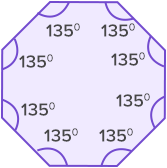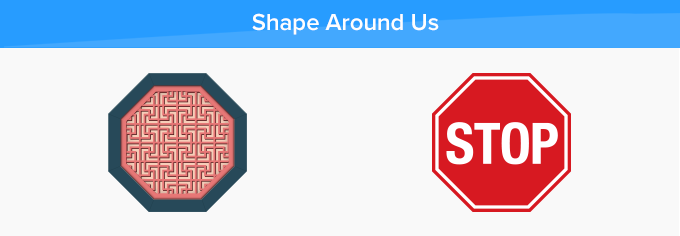# Octagon - Definition with Examples

The Complete K-5 Math Learning Program Built for Your Child

• 40 Million Kids

Loved by kids and parent worldwide

• 50,000 Schools

Trusted by teachers across schools

• Comprehensive Curriculum

Aligned to Common Core

What is Octagon?

An octagon is a polygon made up of 8 sides. It has eight angles.Octagon = Octa + gon where octa means eight and gon means sides.

There are 20 diagonals in an octagon as shown below.The following figures are not octagons:It is a heptagon as it has seven sides.It is a pentagon as it has five sides.It is a hexagon as it has six sides.It is a quadrilateral as it has four sides.

Angles of an octagon:

An octagon consists of 8 angles. The sum of the angles of an octagon is 1080°.In the given octagon, ∠A + ∠B + ∠C + ∠D + ∠E + ∠F + ∠G + ∠H = 1080°.

An octagon has 8 exterior angles. The sum of the exterior angles of an octagon is 360°.

 Regular octagon: An octagon in which all sides and angles are equal. Each angle of a regular octagon is 135°. Irregular octagon: An octagon in which all sides and all angles are not equal. Measures of angles of an irregular octagon are different but they all sum to 1080°.Real life examples

Some of the most popular examples of the octagon are the STOP signboard and umbrella.Fun Facts about octagon The word ‘Octagon’ has been derived from the Greek word ‘ὀκτάγωνον’ (oktágōnon) which means eight angles. This is how the shape with eight angles was named Octagon. During the 1850s and 1900s, many people started building their homes in the shape of an octagon as it is a symbol that represents rebirth, infinity, regeneration, and transition.

Won Numerous Awards & Honors